# Problem 46087. Investigate the frequency of last non-zero digits of primorials

Cody Problems 46054, 46057, and 46060 involve the primorial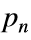#, or the product of prime numbers up to and including the nth prime. In particular, the second of these deals with the last non-zero digit (LNZD) in the primorial. For example, the first eleven primorials are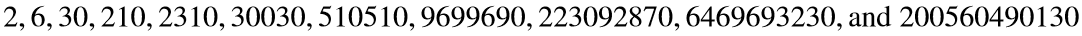After the second primorial, the LNZD is 1, 3, 7, or 9. This observation holds for larger n as well because prime numbers greater than 5 end in 1, 3, 7, or 9 and products of numbers whose LNZDs are 1, 3, 7, or 9 also have a LNZD of 1, 3, 7, or 9.
Mathematicians have determined that the last digits of prime numbers repeat themselves less often than expected. If these digits occurred randomly, 25% of the primes that follow primes ending in a 1 should also end in a 1. However, as verified in Cody Problem 45994, for primes up to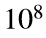, the actual frequency is less than 18%.
What about primorials? What happens when basic multiplication facts interact with the frequency of last digits of primes? Will the frequencies be similarly skewed? Or will they all approach 25%?
Write a function that takes as input an integer n and computes the frequency of the last digits of primorials between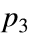# = 30 and#. Return a matrix whose rows correspond to the digits of the first primorial and columns correspond to the digits of the next primorial. Please remember to (a) omit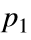# = 2 and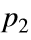# = 6 and (b) account for the primorial following#. For example, if your function is given n = 10, then it should return
0.3333 0.3333 0 0.3333
0.6667 0.3333 0 0
0 1.0000 0 0
0 0 1.0000 0
That is, with n = 10, we consider eight primorials (# through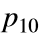#). Of these eight, three have a LNZD of 3 (30, 30030, and 6469693230). Two of those (i.e., 66.7%) are followed by primorials with a LNZD of 1, and one (33.3%) is followed by a primorial with a LNZD of 3.

### Solution Stats

90.91% Correct | 9.09% Incorrect
Last Solution submitted on Apr 30, 2023

### Community Treasure Hunt

Find the treasures in MATLAB Central and discover how the community can help you!

Start Hunting!